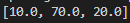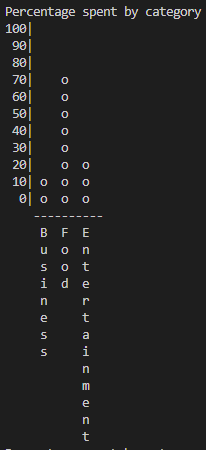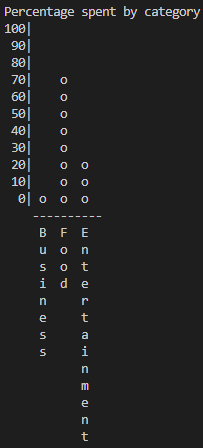# Budget App: Percentage Calculations

Tell us what’s happening:
My calculations are giving results different from intended:

``````def create_spend_chart(categories):
total_withdrawals = list()
for category in categories:
withdrawals = 0
for i in range(1, len(category.ledger)):
withdrawals += category.ledger[i]["amount"]
total_withdrawals.append(withdrawals)

percentages = [round(w/sum(total_withdrawals)*100, -1) for w in total_withdrawals]
print(percentages)
``````

It gives following list as O/P:If I use the above list to plot graph, my o/p is:But, the expected o/p is:As it can be seen that the first graph plots, 10 + 70 + 30 = 100%, but the second graph plots 0 + 70 + 20 = 90% .

So, what should I do?

User Agent is: `Mozilla/5.0 (Windows NT 6.3) AppleWebKit/537.36 (KHTML, like Gecko) Chrome/90.0.4430.85 Safari/537.36`.

Challenge: Budget App

Consider why the expected output only adds up to 90% when the y-axis is in intervals of 10.
Perhaps calculate the exact percentage/value that each column/bar represents?
I don’t remember the exact percentages, but I believe it’s something like 7% + 72% + 21%. The reason you get the percentages 10, 70 and 20 might be because you use real-life math where you would round 7 up to 10 rather than down to 0.

In other words; Consider why you get 7, 72 and 21 to respectively 10, 70 and 20 instead of 0, 70 and 20 like the expected output - and how you could accommodate this in your code!

1 Like

So, instead of using round(), I should just use math.floor() after dividing the percentages by 10?
Thanks!

Yes, that’d be one way to solve the issue, however if I remember correctly, you are not supposed to import any modules in the challenge …

Instead you could consider how to either:
a) Floor it “manually” (without the help of the math module), or
b) Maybe a comparison operator can do the trick - If you store the exact percentage, say ~7%, instead of converting this to 0, why not check if this number is greater than or equal to 0 (yes, so make the `o` in the output here, but when it compares it to 10 it will not print the `o`.
Does that make sense?percentages = [ ((w/sum(total_withdrawals)) * 100) // 10 for w in total_withdrawals]

^ that will make percentages == [0.0,7.0,2.0], right?
Thanks for your help!!Yes, that should floor it without using the math module!1 Like

This topic was automatically closed 182 days after the last reply. New replies are no longer allowed.